##### Quantum Physics For Dummies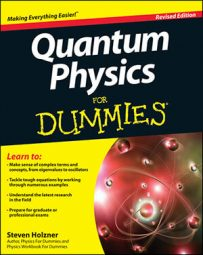In quantum physics, when you have the eigenstates of a system, you can determine the allowable states of the system and the relative probability that the system will be in any of those states.

The commutator of operators A, B is [A, B] = AB – BA, so note that the commutator of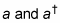is the following: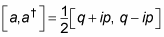This is equal to the following: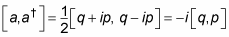This equation breaks down to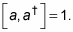And putting together this equation with the Hamiltonian,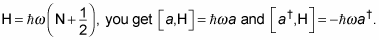Okay, with the commutator relations, you’re ready to go. The first question is: if the energy of state | n > is En, what is the energy of the state a | n >? Well, to find this, rearrange the commutator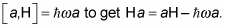Then use this to write the action of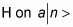like this: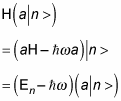So a | n > is also an eigenstate of the harmonic oscillator, with energy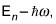not En. That’s why a is called the annihilation or lowering operator: It lowers the energy level of a harmonic oscillator eigenstate by one level.

So what’s the energy level of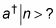You can write that like this: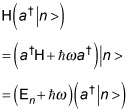All this means that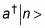is an eigenstate of the harmonic oscillator, with energy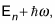not just En — that is, the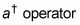raises the energy level of an eigenstate of the harmonic oscillator by one level.

So now you know that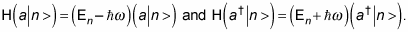You can derive the following from these equations: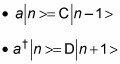C and D are positive constants, but what do they equal? The states |n – 1> and |n + 1> have to be normalized, which means that <n – 1|n – 1> = <n + 1|n + 1> = 1. So take a look at the quantity using the C operator: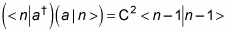And because |n – 1> is normalized, <n – 1|n – 1> = 1: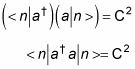But you also know that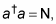the energy level operator, so you get the following equation:

< n | N | n > = C2

N | n > = n | n >, where n is the energy level, so

n < n | n > = C2

However, < n | n > = 1, so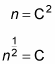This finally tells you, from a | n > = C | n – 1 >, that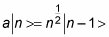That’s cool — now you know how to use the lowering operator, a, on eigenstates of the harmonic oscillator.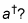First you rearrange the commutator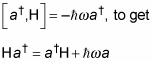Then you follow the same course of reasoning you take with the a operator to show the following: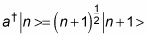So at this point, you know what the energy eigenvalues are and how the raising and lowering operators affect the harmonic oscillator eigenstates.